Nickzom Calculator Calculates Weft Weight in Polymer and Textile Engineering – The Calculator Encyclopedia

Weft is a basic component used in weaving to turn yard or loom into fabric.  The transverse weft is drawn through and inserted over and under the warp. A single thread crossing the warp is called a pick.

According to Wikipedia,

Because the weft does not have to be stretched on a loom the way the warp is it can generally be less strong. It is usually made of spun fibre, originally woolflax and cotton, today often of synthetic fiber such as nylon or rayon.

The weft is threaded through the warp using a “shuttle“, air jets or “rapier grippers”. Hand looms were the original weaver’s tool, with the shuttle being threaded through alternately raised warps by hand.

Nickzom Calculator calculates a bunch of calculations in Polymer and Textile Engineering and shows the steps (workings), formulas and answers. Today, I would showing you with pictures how The Calculator Encyclopedia can calculate the Weft Weight in Kilograms (Kg).

First, you need to have access to the professional version of Nickzom Calculator (Nickzom Calculator+) via any of these channels:

Select Engineering and Click on Open Section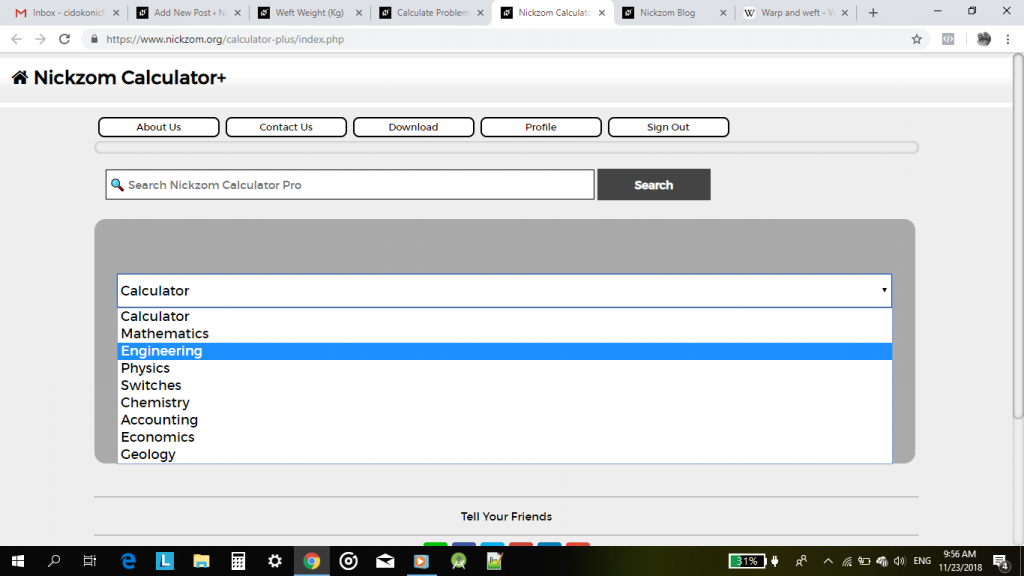A list of Engineering Majors of which its calculations can be handled by the calculator encyclopedia is listed out in alphabetical order. Scroll down and click on Polymer & Textile.The Polymer and Textile Engineering Page has over 200 calculative parameters. Scroll down to Weft Weight (Kg) and click on it.

The Calculator Encyclopedia Calculates the Length of An Open Belt Drive in the Transmission of Power by Belts and Ropes

Calculating the length of an open belt drive is a common thing in Mechanical Engineering. It was of those crucial parameters in the determining the transmission of power by belts and ropes.

In our world today, there are a lot of mechanical engines making use of belts and I believe as a Mechanical Engineer having access to Nickzom Calculator+ is one of the best tools to make lifer easier, faster and simpler.

Nickzom Calculator+ (Professional Version) – The Calculator Encyclopedia can solve over 10,000 calculations for a day to day Mechanical Engineer and today I would show you the steps on how to get the answer for the length of an open belt drive using Nickzom Calculator.

First and Foremost, you need to have access to Nickzom Calculator+ via any of these means:

You must also know that to get the length of an open belt drive there are 3 major parameters you need which are:

• Distance between the Centres of the Larger and Smaller Pullerys
• Radius of the Larger Pulley
• Radius of the Smaller Pulley

Once, you have access to the professional version of Nickzom Calculator via any means, move over to the calculator map, then scroll to the Engineering section and click on Mechanical.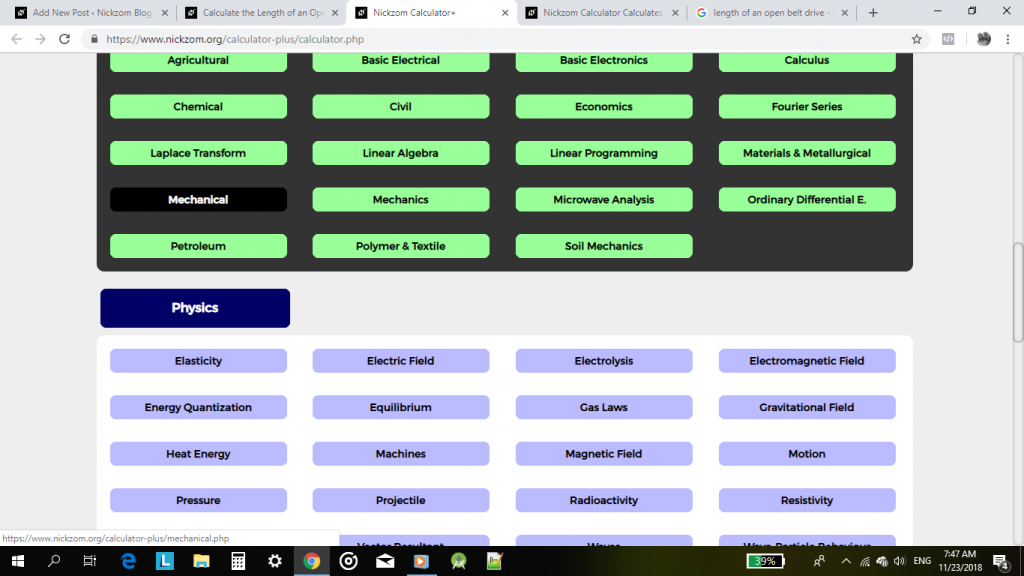On clicking on Mechanical, you see a bunch of major topics that can be solved by Nickzom Calculator. Click on Transmission of Power by Belts and Ropes.Nickzom Calculator Calculates the Due Date of a Woman’s Pregnancy From Your Last Period

According to YourDueDate.com,

Your due date is calculated by adding 280 days (40 weeks) to the first day of your last menstrual period (assuming a 28 day cycle).

Note that your menstrual period and ovulation are counted as the first two weeks of pregnancy. If you deliver on your due date, your baby is actually only 38 weeks old, not 40.

According to BabyCentre,

Unless you’ve been tracking your ovulation, there’s no way to know for sure exactly when you ovulated and conceived. That’s why your due date is calculated based on the date of your last period instead.

Nickzom calculator is capable of estimating your due date for you in a matter of seconds. All you need to know to get your due date are:

• The first day of your last period
• Your average length of cycles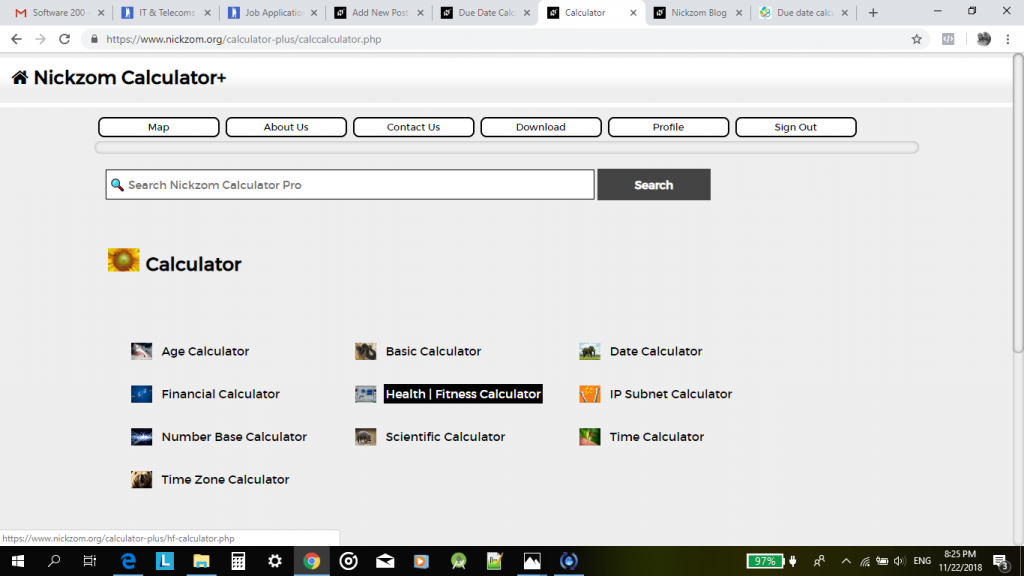Click on Health | Fitness Calculator

Mathematical Ratios Can Be Solved By Nickzom Calculator – The Calculator Encyclopedia

According to Math Is Fun,

A ratio says how much of one thing there is compared to another thing.

According to Wikipedia,

A ratio may be considered as an ordered pair of numbers, as a fraction with the first number in the numerator and the second as denominator, or as the value denoted by this fraction. Ratios of counts, given by (non-zero) natural numbers, denote positive rational numbers, and as such may occasionally result in natural numbers.

According to WikiHow,

Ratios are mathematical expressions that compare two or more numbers. They can compare absolute quantities and amounts or can be used to compare portions of a larger whole. Ratios can be calculated and written in several different ways, but the principles guiding the use of ratios are universal to all.

Nickzom Calculator+ (Professional Version) – The Calculator Encyclopedia solves ratio in mathematics for any number of fractional parts.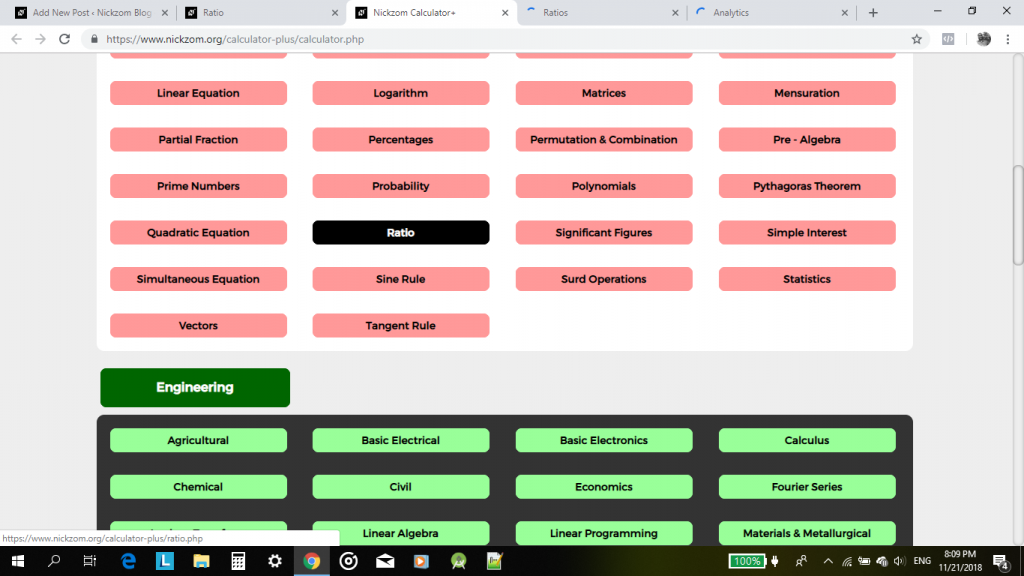For the calculator encyclopedia to be able to solve and present you a ratio. you must have to know the number of fractional parts you desire to compute the ratio for.

The Top 10 Calculator Apps on Google Play Store for Android Devices

1. NICKZOM CALCULATOR    Free/ \$25.99
The calculator encyclopedia consists of two calculators, the Nickzom and Nickzom+ calculators. The Nickzom calculator is an app that solves calculations and shows the steps on Mathematics, Physics, Engineering and Unit Conversions for quick and easy understanding.The goal of this math app is to provide one with solutions and steps to his or her calculations. Alongside a basic and scientific calculator. In Mathematics, Engineering, Physics and Unit Conversions, this app presents the formula, equations, parameters and operations, steps and answer to your question. The Nickzom+ has added calculator features for Health/fitness, Age, Basic, BMI, Date, Financial, Time, Time Zone, IP Subnet, Number Base and Scientific calculator and 30+ Unit Converters,
A very lovely app, simple UI for everyone. it’s like an answer to all your problems.

2. CALCU  Free/\$1.99
Calcu is one of the most popular calculator apps for Android. It features all of the basics. Some other features include a calculation history, memory keys, various customization features, themes, and gesture controls.It’s not a full scientific calculator, but it does have enough functions that goes beyond what you normally find in basic calculators with nice themes to suit your style. So if you’re looking for something a bit more than the basics, this works. The free version of the app contains ads.

3. GOOGLE CALCULATOR Free
The Google Calculator provides simple and advanced mathematical functions in a beautifully designed app. Performs basic calculations such as addition, subtraction, multiplication, and division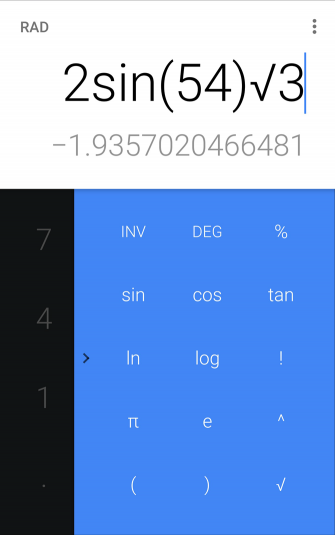You can get some more complex functions by swiping in from the right side, but it’s still rather limited, with a set of functions like trigonometry, logarithm etc. which are available. The other major feature is the three-dot tab. You can tap the top of the screen to reveal a three-dot menu that allows you to change decimals to fractions—and that’s about it. There’s no history or graphing.

4. DEMOS GRAPHING CALCULATOR   Free
Demos Graphing Calculator is a good graphing calculator app substitute for the more popular handheld graphing calculators. It can do the basic stuff like most calculator apps. There is also a variety of scientific calculator options available. It can also do statistics, tables and more. Graphs are interactive with tons of data to share.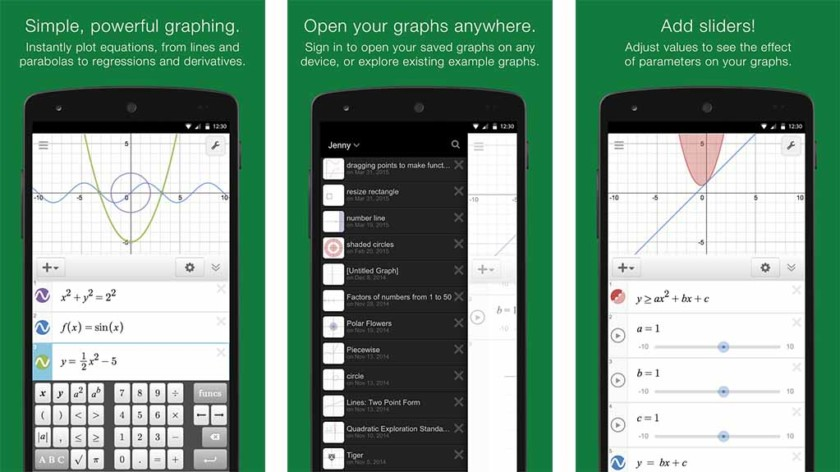Additionally, tables and statistics  can be customized. It will work well for most types of academia. It’s completely free with no in-app purchases.

5. DIGITALCHEMY CALCULATOR    Free/\$1.99
Digitalchemy has developed three calculator apps on Google play store Calculator plus, Fraction calculator and Artful calculator. The Calculator plus and the Artful calculator are basic calculators but with the latter having more themes. The Fraction calculator focuses more on fraction, carrying out all calculations in fractions. The Calculator plus is perfect for shopping, doing homework, balancing checkbooks, or even calculating taxes.
They are easy to use and beautifully designed to do things better than your phone or handheld calculator ever did.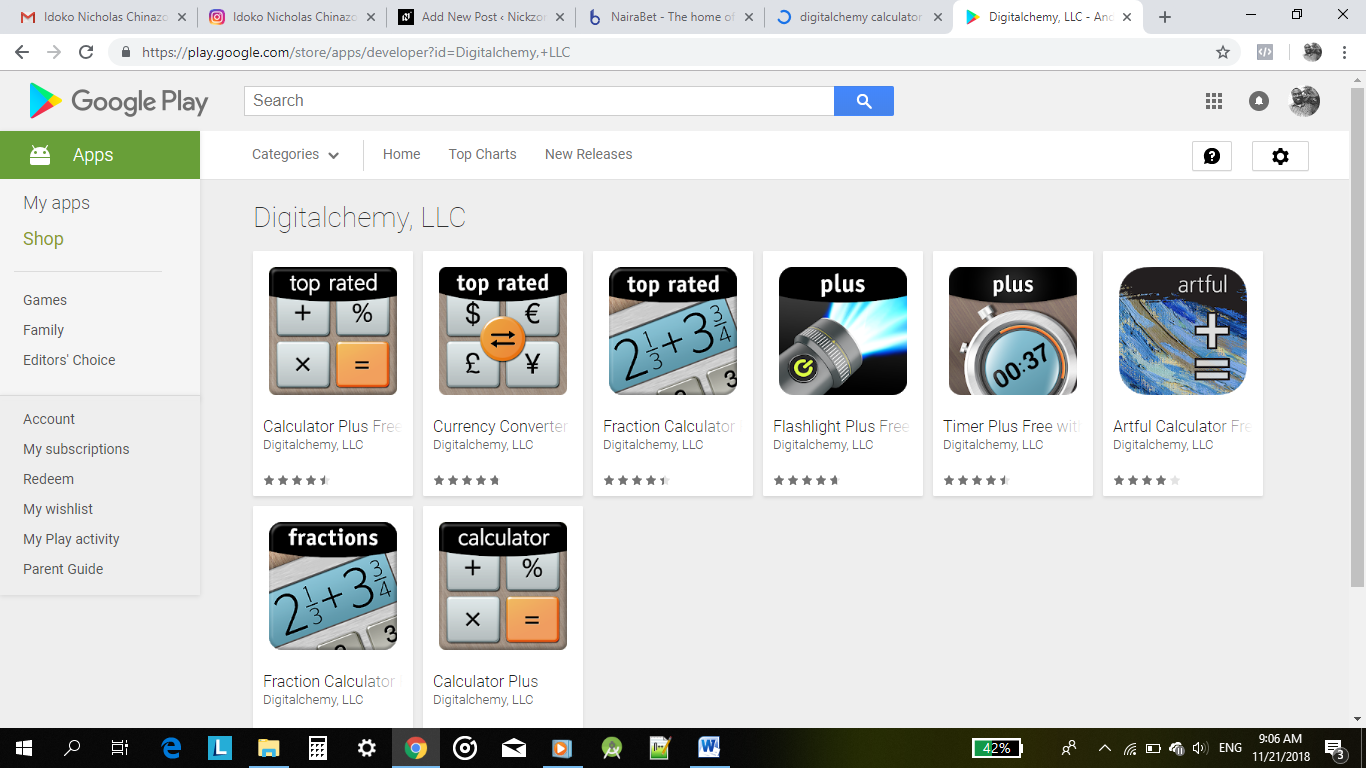6. GRAPHING CALCULATOR BY MATHLAB   Free/\$6.99
Graphing Calculator by Mathlab is a scientific graphing calculator integrated with algebra and is an indispensable mathematical tool for students from high school to those in college or graduate school, or just anyone who needs more than what a basic calculator offers. It is designed to replace bulky and costly handheld graphing calculators and works on virtually any Android device or tablet. Nice 3D graphical view for better representation. Good for academic work.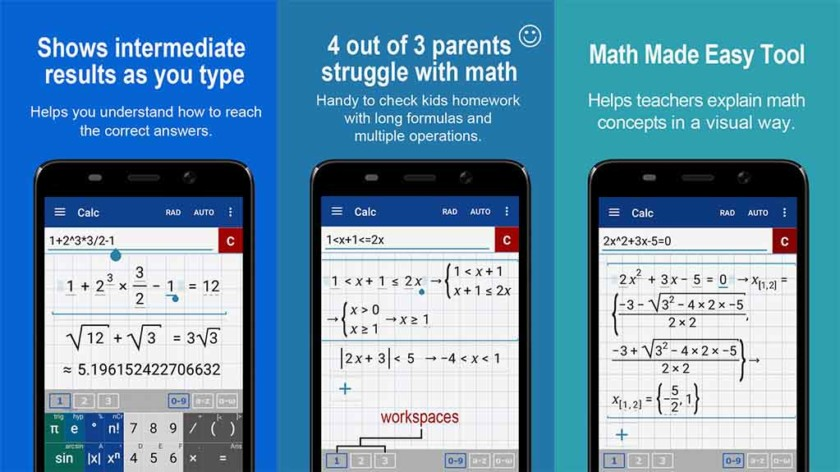7. FINANCIAL CALCULATOR    Free
Financial Calculators is a series of calculators to help you make sense and keep track of your finances. It features various modes that can help you easily calculate anything from Return of Investments(ROI), to loan interest and even taxes. There have been reported issues with a few of the modes, but the vast majority of them work pretty well. Anyone in need of keeping up with their finances should check this out. It’s completely free with no in-app purchases. It does have ads.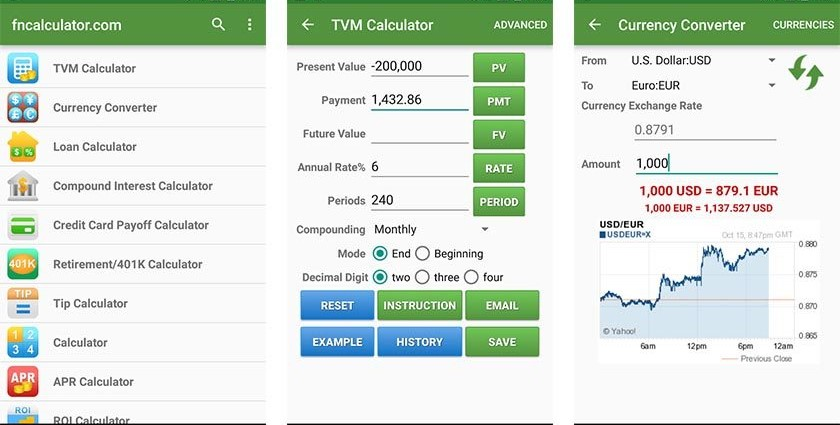8. HiPER SCIENTIFIC CALCULATOR    Free/\$2.99
The calculator has 15 digits of significand and 3 digits of exponent in its free version and up to 100 digits of significand and 9 digits of exponent in HiPER Calc Pro. It has most of the basic scientific calculator functions, a built-in unit converter with over 200 units, and even some obscure features like a random number generator, permutations, etc.  It detects repeating decimals and numbers can also be entered as fractions or converted to fractions. The Pro version has the “expression” mode in which you can write expressions in a natural way and watch your calculations. The result is displayed as a number, simplified expression etc.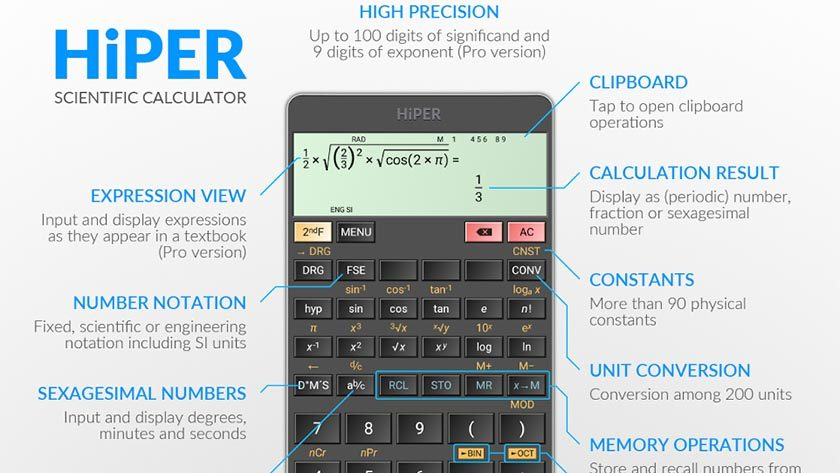9. REALCALC
The RealCalc Plus is the Pro version of the RealCalc calculator app,designed to look and operate exactly like a real hand-held calculator. It has all the standard scientific functions plus fractions, degrees/minutes/seconds, history, memories, customizable unit conversions and constants. You can choose from a number of display styles and formats. It also supports binary, octal and hexadecimal calculations and has an optional RPN mode. RealCalc Plus is easy to use, but has full help included in the app.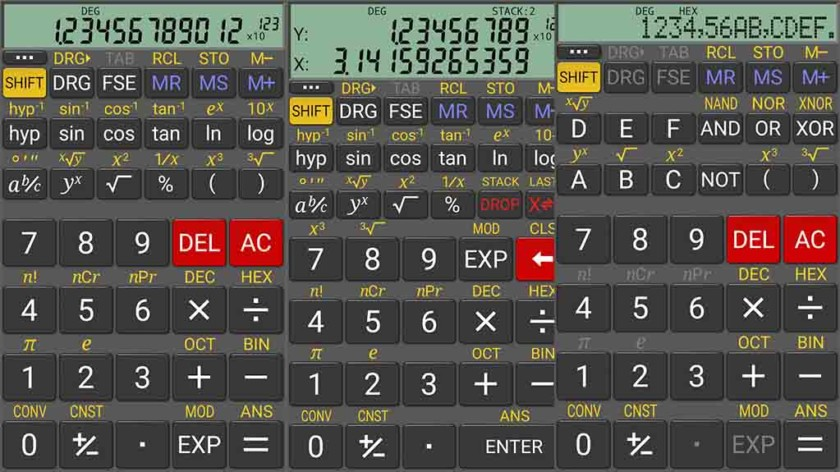10. CALCULATOR++    Free/\$4.00
The Calculator++ offers more than the basic calculator. This app has both a Simple and an Engineer mode. It supports a wide variety of functions, a history of everything you’ve calculated, and it can even create graphs.

One cool feature is the swipe buttons. Swipe up on a button to activate the above option, and swipe down to activate the option below. It’s also customizable, with several different theme options available. Along with a widget for your home screen, it includes a floating window mode.
This app does contain ads. You can get rid of ads for \$4. Overall, it strikes a nice balance between being simple and functional.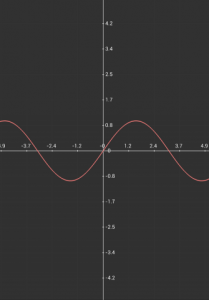If we missed any of your best calculator apps for Android, you can tell us about them in the comments section below.

The Calculator Encyclopedia Solves For Input and Output Reflection Coefficients, Power Gains and Mismatch Factors of S Parameters in Microwave Analysis

The knowledge of microwave analysis is a very added advantage for any Electronic Engineer out there. It makes one better understand the networking system of a two port in relation to S parameter.

According to Wikipedia, the S Parameters describe the electrical behavior of linear electrical networks when undergoing various steady state stimuli by electrical signals.

Luckily, Nickzom Calculator, the calculator encyclopedia is happy to take care of all calculations dealing with S Parameter (Two Port Network) for you and also show you a step by step guide (workings) on how to solve this problem.

For the purpose of this post, I would show you how Nickzom Calculator solves for the following:

• Input and Output Reflection Coefficients
• Various Power Gains
• Mismatch Factors

First of all, you have to have access to this awesome software package.

One can access Nickzom Calculator+ (Professional Version) – The Calculator Encyclopedia via any of these channels:

Once you have got access to the software package, please proceed to the Calculator Map then scroll to Engineering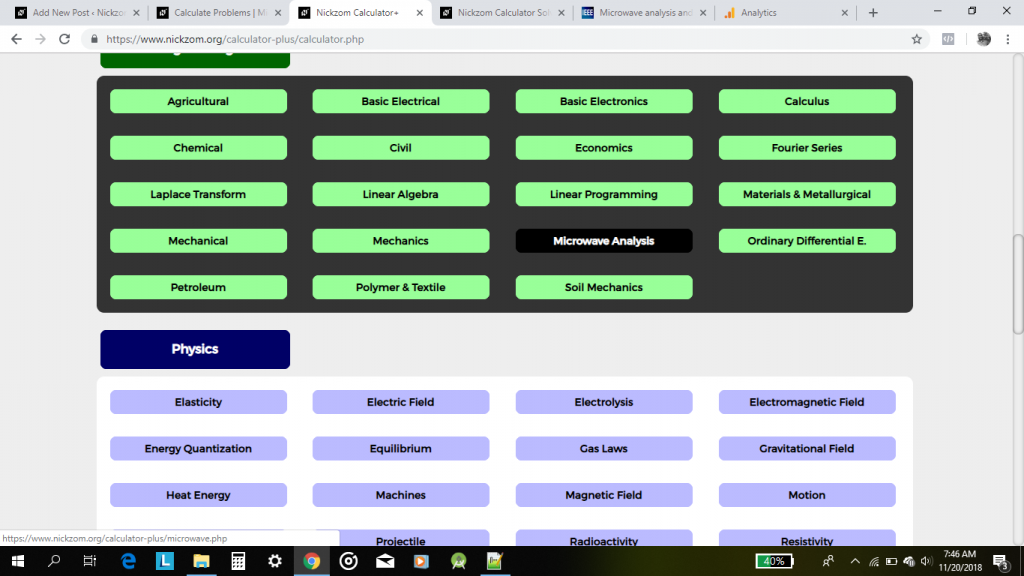Nickzom Calculator Solves Formation Factor of Well Logging in Petroleum Engineering

According to Schlumberger OilField Glossary,

The ratio of the resistivity of a rock filled with water (Ro) to the resistivity of that water (Rw).

One can access Nickzom Calculator+ (Professional Version) – The Calculator Encyclopedia via any of these channels: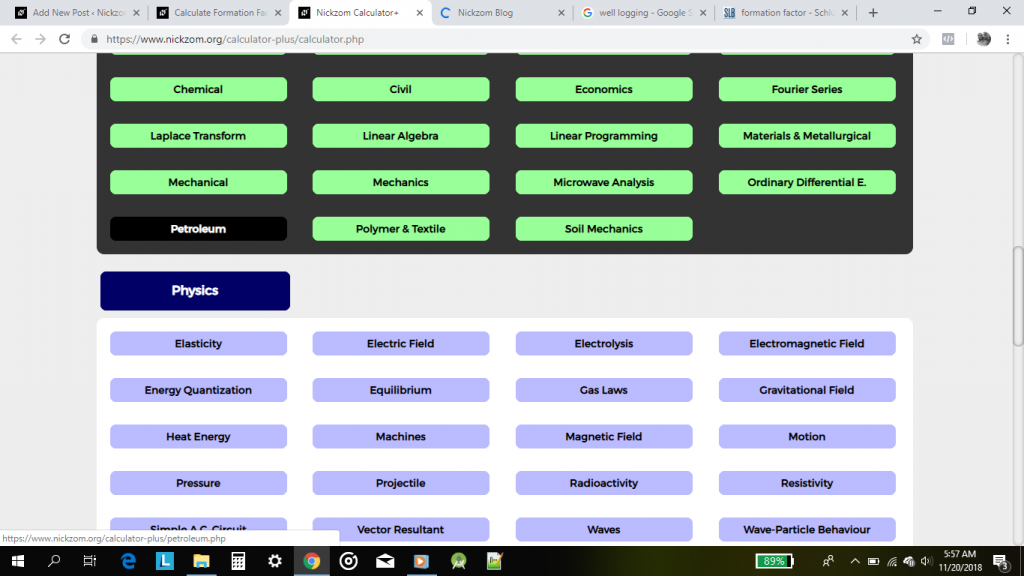On selecting Petroleum, a bunch of links to major topics in Petroleum Engineering is displayed. Scroll down to Well Logging and click on it.

According to Wikipedia,

Well logging, also known as borehole logging is the practice of making a detailed record (a well log) of the geologic formations penetrated by a borehole. The log may be based either on visual inspection of samples brought to the surface (geological logs) or on physical measurements made by instruments lowered into the hole(geophysical logs).The Calculator Encyclopedia Converts Units of Sound

According to Wikipedia,

The decibel (symbol: dB) is a unit of measurement used to express the ratio of one value of a physical property to another on a logarithmic scale. It can be used to express a change in value (e.g., +1 dB or −1 dB) or an absolute value. In the latter case, it expresses the ratio of a value to a reference value; when used in this way, the decibel symbol should be appended with a suffix that indicates the reference value, or some other property. For example, if the reference value is 1 volt, then the suffix is “V” (e.g., “20 dBV”), and if the reference value is one milliwatt, then the suffix is “m” (e.g., “20 dBm”).

Also, according to Wikipedia,

The neper (symbol: Np) is a logarithmic unit for ratios of measurements of physical field and power quantities, such as gain and loss of electronic signals. The unit’s name is derived from the name of John Napier, the inventor of logarithms. As is the case for the decibel and bel, the neper is a unit defined in the international standard ISO 80000. It is not part of the International System of Units (SI), but is accepted for use alongside the SI.

Nickzom Calculator+ (Professional Version) – The Calculator Encyclopedia converts the units of sounds and at the moment, it converts three of them which are:

• Bels
• Decibels
• Neper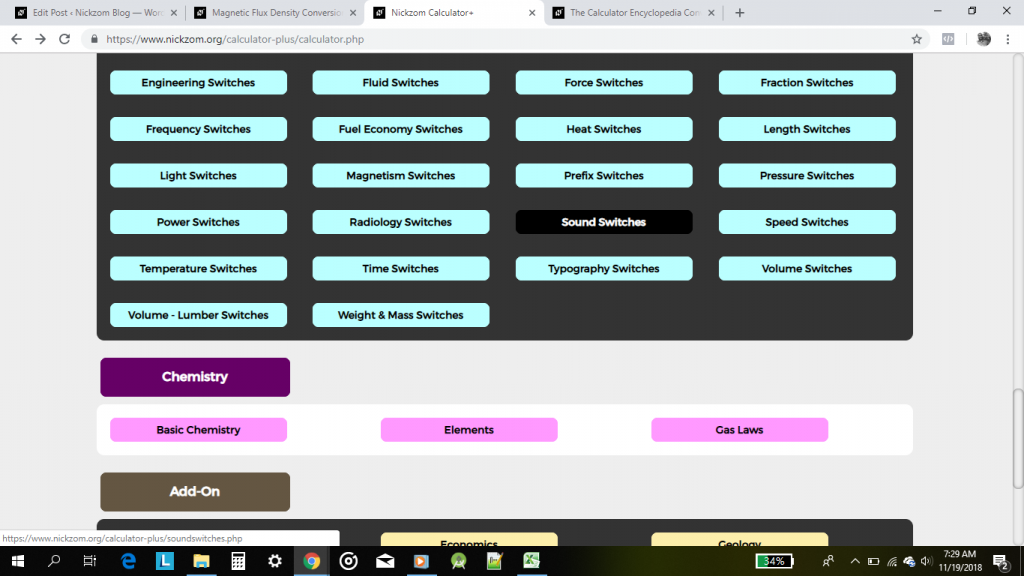Click on Sound Switches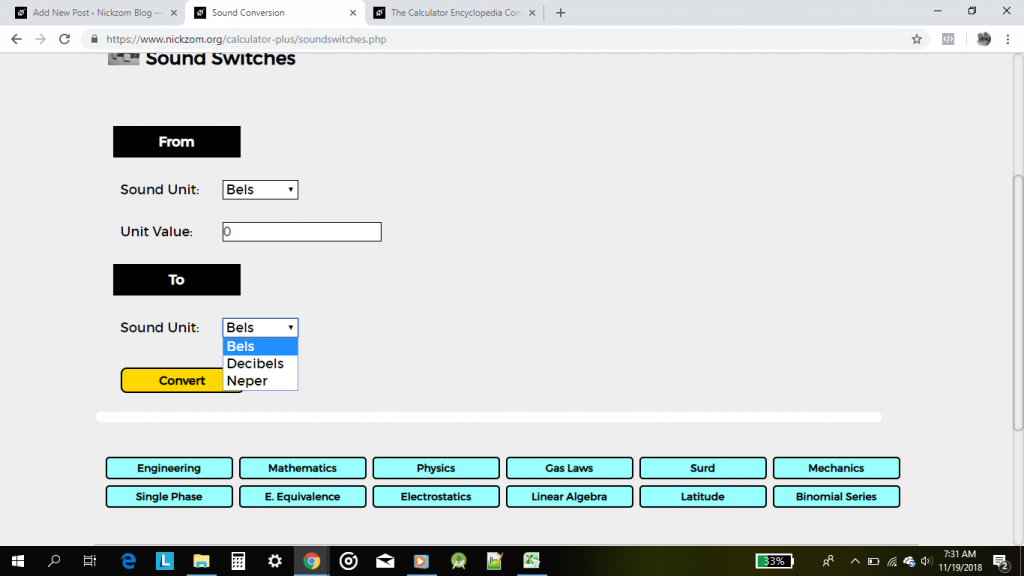For the purpose of this post, we are to convert 45 Decibels to Neper

The Calculator Encyclopedia Converts Magnetic Flux Density Units on Magnetism Unit Conversions

According to Mini Physics,

Magnetic flux density (B) is defined as the force acting per unit current per unit length on a wire placed at right angles to the magnetic field.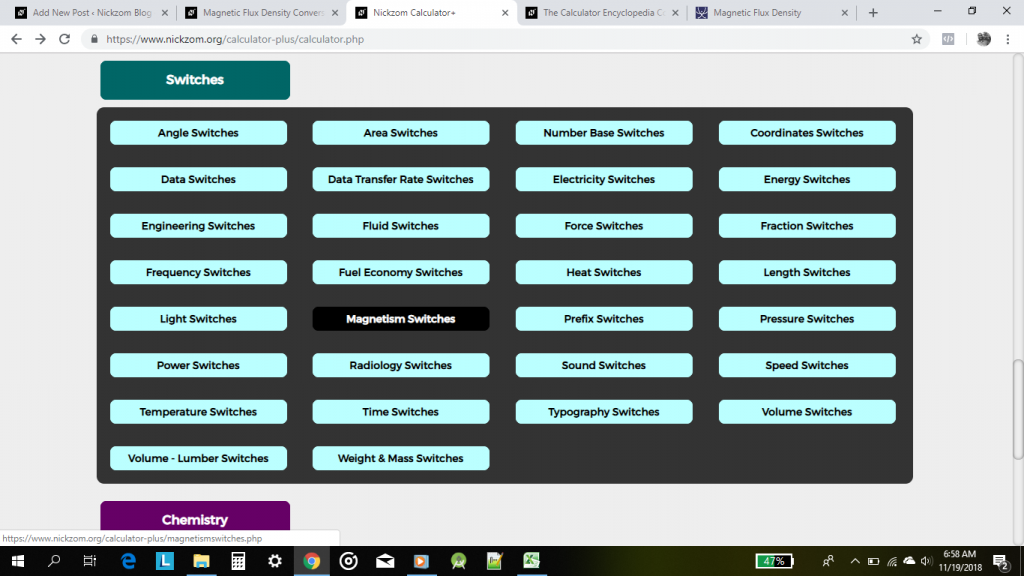Nickzom Calculator converts 11 units of magnetic flux density such as:

• Tesla
• Weber/sq. meter
• Weber/sq. centimeter
• Weber/sq. inch
• Maxwell/sq. meter
• Maxwell/sq. centimeter
• Maxwell/sq. inch
• Gauss
• Lines/sq. centimeter
• Lines/sq. inch
• Gamma

On Clicking on Magnetism Switches, there are 4 major magnetism unit conversion sections such as:

• Magnetomotive Force Switches
• Magnetic Field Strength Switches
• Magnetic Flux Switches
• Magnetic Flux Density Switches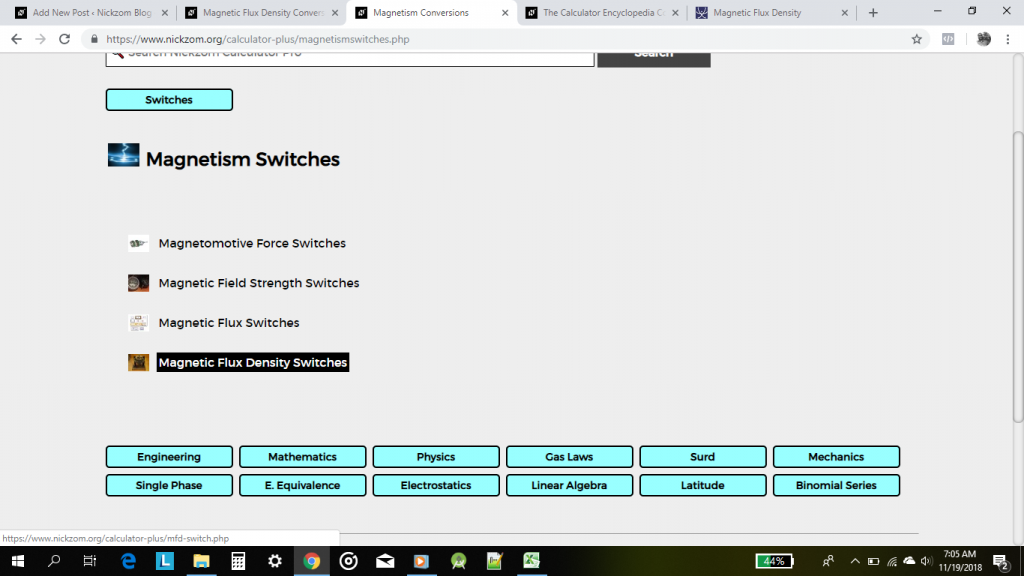The Calculator Encyclopedia Converts Radiation- Exposure Units in Radiology Unit Conversion

According to Wikipedia,

Radiation exposure is a measure of the ionization of air due to ionizing radiation from photons; that is, gamma rays and X-rays. It is defined as the electric charge freed by such radiation in a specified volume of air divided by the mass of that air.

Nickzom Calculator+ (Professional Version) – The Calculator Encyclopedia converts 7 units of Radiation Exposure which are:

• Coulombs/kilogram
• Millicoulombs/kilogram
• Microcoulombs/kilogram
• Roentgen
• Tissue Roentgen
• Parker
• Rep

One can access Nickzom Calculator+ (Professional Version) – The Calculator Encyclopedia via any of these channels:

For the purpose of this post, I will display two different radiation – exposure unit conversions which are:

• Coulombs/kilogram to Tissue Roentgen
• Roentgen to Parker

Let’s begin with Coulombs/kilogram to Tissue Roentgen

Access the Calculator Map, scroll to Switches Section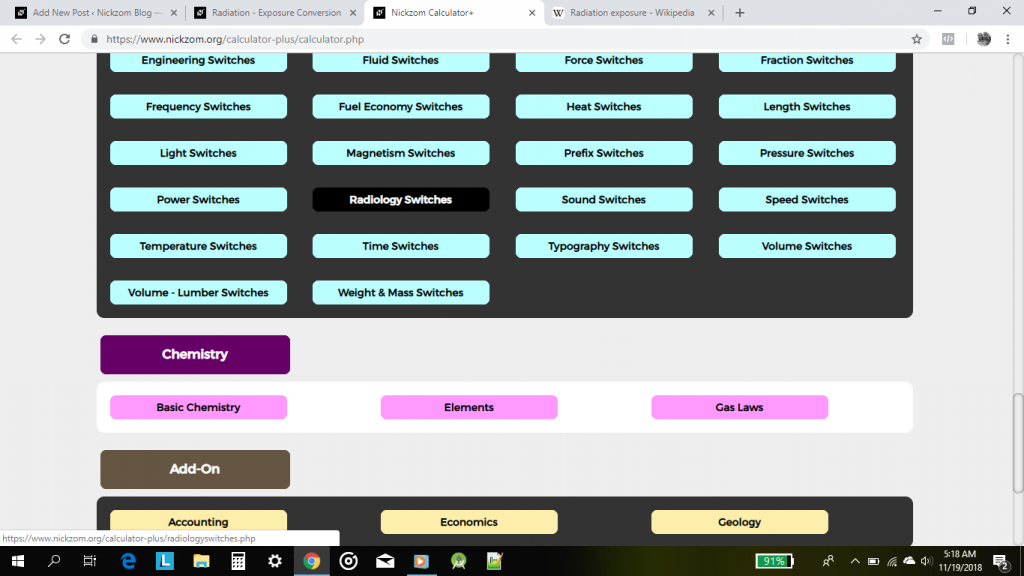Now, click on Radiology Switches, this would display four unit conversions under Radiology which are: